# A Beginner's Guide to Neural Networks and Deep Learning

Contents

## Neural Network Definition

Neural networks are a set of algorithms, modeled loosely after the human brain, that are designed to recognize patterns. They interpret sensory data through a kind of machine perception, labeling or clustering raw input. The patterns they recognize are numerical, contained in vectors, into which all real-world data, be it images, sound, text or time series, must be translated.

Neural networks help us cluster and classify. You can think of them as a clustering and classification layer on top of the data you store and manage. They help to group unlabeled data according to similarities among the example inputs, and they classify data when they have a labeled dataset to train on. (Neural networks can also extract features that are fed to other algorithms for clustering and classification; so you can think of deep neural networks as components of larger machine-learning applications involving algorithms for reinforcement learning, classification and regression.)

Artificial neural networks are the foundation of large-language models (LLMS) used by chatGPT, Microsoft’s Bing, Google’s Bard and Meta’s Llama.

What kind of problems does deep learning solve, and more importantly, can it solve yours? To know the answer, you need to ask a few questions:

• What outcomes do I care about? In a classification problem, those outcomes are labels that could be applied to data: for example, `spam` or `not_spam` in an email filter, `good_guy` or `bad_guy` in fraud detection, `angry_customer` or `happy_customer` in customer relationship management. Other types of problems include anomaly detection (useful in fraud detection and predictive maintenance of manufacturing equipment), and clustering, which is useful in recommendation systems that surface similarities.

• Do I have the right data? For example, if you have a classification problem, you’ll need labeled data. Is the dataset you need publicly available, or can you create it (with a data annotation service like Scale or AWS Mechanical Turk)? In this example, spam emails would be labeled as spam, and the labels would enable the algorithm to map from inputs to the classifications you care about. You can’t know that you have the right data until you get your hands on it. If you are a data scientist working on a problem, you can’t trust anyone to tell you whether the data is good enough. Only direct exploration of the data will answer this question.

### Working on a New AI Startup?

Find Out How Page One Can Support You

## A Few Concrete Examples

Deep learning maps inputs to outputs. It finds correlations. It is known as a “universal approximator”, because it can learn to approximate an unknown function `f(x) = y` between any input `x` and any output `y`, assuming they are related at all (by correlation or causation, for example). In the process of learning, a neural network finds the right `f`, or the correct manner of transforming `x` into `y`, whether that be `f(x) = 3x + 12` or `f(x) = 9x - 0.1`. Here are a few examples of what deep learning can do.

### Classification

All classification tasks depend upon labeled datasets; that is, humans must transfer their knowledge to the dataset in order for a neural network to learn the correlation between labels and data. This is known as supervised learning.

• Detect faces, identify people in images, recognize facial expressions (angry, joyful)
• Identify objects in images (stop signs, pedestrians, lane markers…)
• Recognize gestures in video
• Detect voices, identify speakers, transcribe speech to text, recognize sentiment in voices
• Classify text as spam (in emails), or fraudulent (in insurance claims); recognize sentiment in text (customer feedback)

Any labels that humans can generate, any outcomes that you care about and which correlate to data, can be used to train a neural network.

### Clustering

Clustering or grouping is the detection of similarities. Deep learning does not require labels to detect similarities. Learning without labels is called unsupervised learning. Unlabeled data is the majority of data in the world. One law of machine learning is: the more data an algorithm can train on, the more accurate it will be. Therefore, unsupervised learning has the potential to produce highly accurate models.

• Search: Comparing documents, images or sounds to surface similar items.
• Anomaly detection: The flipside of detecting similarities is detecting anomalies, or unusual behavior. In many cases, unusual behavior correlates highly with things you want to detect and prevent, such as fraud.

### Predictive Analytics: Regressions

With classification, deep learning is able to establish correlations between, say, pixels in an image and the name of a person. You might call this a static prediction. By the same token, exposed to enough of the right data, deep learning is able to establish correlations between present events and future events. It can run regression between the past and the future. The future event is like the label in a sense. Deep learning doesn’t necessarily care about time, or the fact that something hasn’t happened yet. Given a time series, deep learning may read a string of number and predict the number most likely to occur next.

• Hardware breakdowns (data centers, manufacturing, transport)
• Health breakdowns (strokes, heart attacks based on vital stats and data from wearables)
• Customer churn (predicting the likelihood that a customer will leave, based on web activity and metadata)
• Employee turnover (ditto, but for employees)

The better we can predict, the better we can prevent and pre-empt. As you can see, with neural networks, we’re moving towards a world of fewer surprises. Not zero surprises, just marginally fewer. We’re also moving toward a world of smarter agents that combine neural networks with other algorithms like reinforcement learning to attain goals.

With that brief overview of deep learning use cases, let’s look at what neural nets are made of.

## Neural Network Elements

Deep learning is the name we use for “stacked neural networks”; that is, networks composed of several layers.

The layers are made of nodes. A node is just a place where computation happens, loosely patterned on a neuron in the human brain, which fires when it encounters sufficient stimuli. A node combines input from the data with a set of coefficients, or weights, that either amplify or dampen that input, thereby assigning significance to inputs with regard to the task the algorithm is trying to learn; e.g. which input is most helpful is classifying data without error? These input-weight products are summed and then the sum is passed through a node’s so-called activation function, to determine whether and to what extent that signal should progress further through the network to affect the ultimate outcome, say, an act of classification. If the signals passes through, the neuron has been “activated.”

Here’s a diagram of what one node might look like.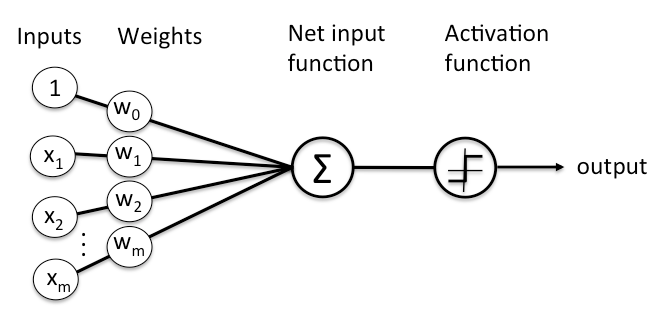A node layer is a row of those neuron-like switches that turn on or off as the input is fed through the net. Each layer’s output is simultaneously the subsequent layer’s input, starting from an initial input layer receiving your data.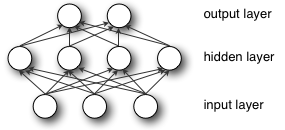Pairing the model’s adjustable weights with input features is how we assign significance to those features with regard to how the neural network classifies and clusters input.

## Key Concepts of Deep Neural Networks

Deep-learning networks are distinguished from the more commonplace single-hidden-layer neural networks by their depth; that is, the number of node layers through which data must pass in a multistep process of pattern recognition.

Earlier versions of neural networks such as the first perceptrons were shallow, composed of one input and one output layer, and at most one hidden layer in between. More than three layers (including input and output) qualifies as “deep” learning. So deep is not just a buzzword to make algorithms seem like they read Sartre and listen to bands you haven’t heard of yet. It is a strictly defined term that means more than one hidden layer.

In deep-learning networks, each layer of nodes trains on a distinct set of features based on the previous layer’s output. The further you advance into the neural net, the more complex the features your nodes can recognize, since they aggregate and recombine features from the previous layer.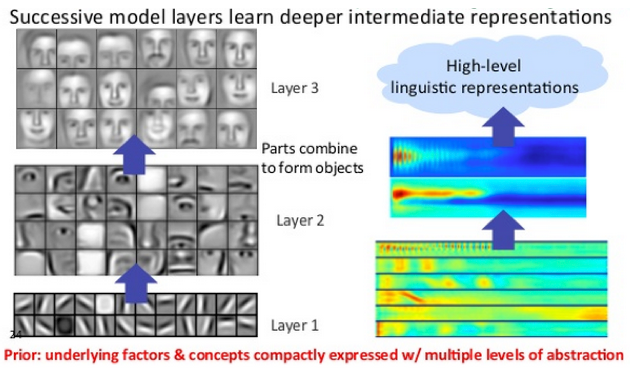This is known as feature hierarchy, and it is a hierarchy of increasing complexity and abstraction. It makes deep-learning networks capable of handling very large, high-dimensional data sets with billions of parameters that pass through nonlinear functions.

Above all, these neural nets are capable of discovering latent structures within unlabeled, unstructured data, which is the vast majority of data in the world. Another word for unstructured data is raw media; i.e. pictures, texts, video and audio recordings. Therefore, one of the problems deep learning solves best is in processing and clustering the world’s raw, unlabeled media, discerning similarities and anomalies in data that no human has organized in a relational database or ever put a name to.

For example, deep learning can take a million images, and cluster them according to their similarities: cats in one corner, ice breakers in another, and in a third all the photos of your grandmother. This is the basis of so-called smart photo albums.

Now apply that same idea to other data types: Deep learning might cluster raw text such as emails or news articles. Emails full of angry complaints might cluster in one corner of the vector space, while satisfied customers, or spambot messages, might cluster in others. This is the basis of various messaging filters, and can be used in customer-relationship management (CRM). The same applies to voice messages.

With time series, data might cluster around normal/healthy behavior and anomalous/dangerous behavior. If the time series data is being generated by a smart phone, it will provide insight into users’ health and habits; if it is being generated by an autopart, it might be used to prevent catastrophic breakdowns.

Deep-learning networks perform automatic feature extraction without human intervention, unlike most traditional machine-learning algorithms. Given that feature extraction is a task that can take teams of data scientists years to accomplish, deep learning is a way to circumvent the chokepoint of limited experts. It augments the powers of small data science teams, which by their nature do not scale.

When training on unlabeled data, each node layer in a deep network learns features automatically by repeatedly trying to reconstruct the input from which it draws its samples, attempting to minimize the difference between the network’s guesses and the probability distribution of the input data itself. Restricted Boltzmann machines, for examples, create so-called reconstructions in this manner.

In the process, these neural networks learn to recognize correlations between certain relevant features and optimal results – they draw connections between feature signals and what those features represent, whether it be a full reconstruction, or with labeled data.

A deep-learning network trained on labeled data can then be applied to unstructured data, giving it access to much more input than machine-learning nets. This is a recipe for higher performance: the more data a net can train on, the more accurate it is likely to be. (Bad algorithms trained on lots of data can outperform good algorithms trained on very little.) Deep learning’s ability to process and learn from huge quantities of unlabeled data give it a distinct advantage over previous algorithms.

Deep-learning networks end in an output layer: a logistic, or softmax, classifier that assigns a likelihood to a particular outcome or label. We call that predictive, but it is predictive in a broad sense. Given raw data in the form of an image, a deep-learning network may decide, for example, that the input data is 90 percent likely to represent a person.

## Example: Feedforward Networks

Our goal in using a neural net is to arrive at the point of least error as fast as possible. We are running a race, and the race is around a track, so we pass the same points repeatedly in a loop. The starting line for the race is the state in which our weights are initialized, and the finish line is the state of those parameters when they are capable of producing sufficiently accurate classifications and predictions.

The race itself involves many steps, and each of those steps resembles the steps before and after. Just like a runner, we will engage in a repetitive act over and over to arrive at the finish. Each step for a neural network involves a guess, an error measurement and a slight update in its weights, an incremental adjustment to the coefficients, as it slowly learns to pay attention to the most important features.

A collection of weights, whether they are in their start or end state, is also called a model, because it is an attempt to model data’s relationship to ground-truth labels, to grasp the data’s structure. Models normally start out bad and end up less bad, changing over time as the neural network updates its parameters.

This is because a neural network is born in ignorance. It does not know which weights and biases will translate the input best to make the correct guesses. It has to start out with a guess, and then try to make better guesses sequentially as it learns from its mistakes. (You can think of a neural network as a miniature enactment of the scientific method, testing hypotheses and trying again – only it is the scientific method with a blindfold on. Or like a child: they are born not knowing much, and through exposure to life experience, they slowly learn to solve problems in the world. For neural networks, data is the only experience.)

Here is a simple explanation of what happens during learning with a feedforward neural network, the simplest architecture to explain.

Input enters the network. The coefficients, or weights, map that input to a set of guesses the network makes at the end.

``````input * weight = guess
``````

Weighted input results in a guess about what that input is. The neural then takes its guess and compares it to a ground-truth about the data, effectively asking an expert “Did I get this right?”

``````ground truth - guess = error
``````

The difference between the network’s guess and the ground truth is its error. The network measures that error, and walks the error back over its model, adjusting weights to the extent that they contributed to the error.

``````error * weight's contribution to error = adjustment
``````

The three pseudo-mathematical formulas above account for the three key functions of neural networks: scoring input, calculating loss and applying an update to the model – to begin the three-step process over again. A neural network is a corrective feedback loop, rewarding weights that support its correct guesses, and punishing weights that lead it to err.

Let’s linger on the first step above.

### Multiple Linear Regression

Despite their biologically inspired name, artificial neural networks are nothing more than math and code, like any other machine-learning algorithm. In fact, anyone who understands linear regression, one of first methods you learn in statistics, can understand how a neural net works. In its simplest form, linear regression is expressed as

``````    Y_hat = bX + a
``````

where `Y_hat` is the estimated output, `X` is the input, `b` is the slope and `a` is the intercept of a line on the vertical axis of a two-dimensional graph. (To make this more concrete: `X` could be radiation exposure and `Y` could be the cancer risk; `X` could be daily pushups and `Y_hat` could be the total weight you can benchpress; `X` the amount of fertilizer and `Y_hat` the size of the crop.) You can imagine that every time you add a unit to `X`, the dependent variable `Y_hat` increases proportionally, no matter how far along you are on the X axis. That simple relation between two variables moving up or down together is a starting point.

The next step is to imagine multiple linear regression, where you have many input variables producing an output variable. It’s typically expressed like this:

``````    Y_hat = b_1*X_1 + b_2*X_2 + b_3*X_3 + a
``````

(To extend the crop example above, you might add the amount of sunlight and rainfall in a growing season to the fertilizer variable, with all three affecting `Y_hat`.)

Now, that form of multiple linear regression is happening at every node of a neural network. For each node of a single layer, input from each node of the previous layer is recombined with input from every other node. That is, the inputs are mixed in different proportions, according to their coefficients, which are different leading into each node of the subsequent layer. In this way, a net tests which combination of input is significant as it tries to reduce error.

Once you sum your node inputs to arrive at `Y_hat`, it’s passed through a non-linear function. Here’s why: If every node merely performed multiple linear regression, `Y_hat` would increase linearly and without limit as the X’s increase, but that doesn’t suit our purposes.

What we are trying to build at each node is a switch (like a neuron…) that turns on and off, depending on whether or not it should let the signal of the input pass through to affect the ultimate decisions of the network.

When you have a switch, you have a classification problem. Does the input’s signal indicate the node should classify it as enough, or not_enough, on or off? A binary decision can be expressed by 1 and 0, and logistic regression is a non-linear function that squashes input to translate it to a space between 0 and 1.

The nonlinear transforms at each node are usually s-shaped functions similar to logistic regression. They go by the names of sigmoid (the Greek word for “S”), tanh, hard tanh, etc., and they shaping the output of each node. The output of all nodes, each squashed into an s-shaped space between 0 and 1, is then passed as input to the next layer in a feed forward neural network, and so on until the signal reaches the final layer of the net, where decisions are made.

The name for one commonly used optimization function that adjusts weights according to the error they caused is called “gradient descent.”

Gradient is another word for slope, and slope, in its typical form on an x-y graph, represents how two variables relate to each other: rise over run, the change in money over the change in time, etc. In this particular case, the slope we care about describes the relationship between the network’s error and a single weight; i.e. that is, how does the error vary as the weight is adjusted.

To put a finer point on it, which weight will produce the least error? Which one correctly represents the signals contained in the input data, and translates them to a correct classification? Which one can hear “nose” in an input image, and know that should be labeled as a face and not a frying pan?

As a neural network learns, it slowly adjusts many weights so that they can map signal to meaning correctly. The relationship between network Error and each of those weights is a derivative, dE/dw, that measures the degree to which a slight change in a weight causes a slight change in the error.

Each weight is just one factor in a deep network that involves many transforms; the signal of the weight passes through activations and sums over several layers, so we use the chain rule of calculus to march back through the networks activations and outputs and finally arrive at the weight in question, and its relationship to overall error.

The chain rule in calculus states that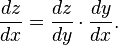In a feedforward network, the relationship between the net’s error and a single weight will look something like this: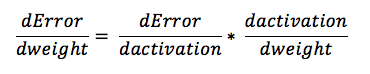That is, given two variables, Error and weight, that are mediated by a third variable, activation, through which the weight is passed, you can calculate how a change in weight affects a change in Error by first calculating how a change in activation affects a change in Error, and how a change in weight affects a change in activation.

The essence of learning in deep learning is nothing more than that: adjusting a model’s weights in response to the error it produces, until you can’t reduce the error any more.

## Logistic Regression

On a deep neural network of many layers, the final layer has a particular role. When dealing with labeled input, the output layer classifies each example, applying the most likely label. Each node on the output layer represents one label, and that node turns on or off according to the strength of the signal it receives from the previous layer’s input and parameters.

Each output node produces two possible outcomes, the binary output values 0 or 1, because an input variable either deserves a label or it does not. After all, there is no such thing as a little pregnant.

While neural networks working with labeled data produce binary output, the input they receive is often continuous. That is, the signals that the network receives as input will span a range of values and include any number of metrics, depending on the problem it seeks to solve.

For example, a recommendation engine has to make a binary decision about whether to serve an ad or not. But the input it bases its decision on could include how much a customer has spent on Amazon in the last week, or how often that customer visits the site.

So the output layer has to condense signals such as \$67.59 spent on diapers, and 15 visits to a website, into a range between 0 and 1; i.e. a probability that a given input should be labeled or not.

The mechanism we use to convert continuous signals into binary output is called logistic regression. The name is unfortunate, since logistic regression is used for classification rather than regression in the linear sense that most people are familiar with. It calculates the probability that a set of inputs match the label.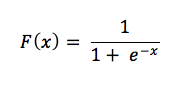Let’s examine this little formula.

For continuous inputs to be expressed as probabilities, they must output positive results, since there is no such thing as a negative probability. That’s why you see input as the exponent of e in the denominator – because exponents force our results to be greater than zero. Now consider the relationship of e’s exponent to the fraction 1/1. One, as we know, is the ceiling of a probability, beyond which our results can’t go without being absurd. (We’re 120% sure of that.)

As the input x that triggers a label grows, the expression e to the x shrinks toward zero, leaving us with the fraction 1/1, or 100%, which means we approach (without ever quite reaching) absolute certainty that the label applies. Input that correlates negatively with your output will have its value flipped by the negative sign on e’s exponent, and as that negative signal grows, the quantity e to the x becomes larger, pushing the entire fraction ever closer to zero.

Now imagine that, rather than having x as the exponent, you have the sum of the products of all the weights and their corresponding inputs – the total signal passing through your net. That’s what you’re feeding into the logistic regression layer at the output layer of a neural network classifier.

With this layer, we can set a decision threshold above which an example is labeled 1, and below which it is not. You can set different thresholds as you prefer – a low threshold will increase the number of false positives, and a higher one will increase the number of false negatives – depending on which side you would like to err.

## Neural Networks & Artificial Intelligence

In some circles, neural networks are synonymous with AI. In others, they are thought of as a “brute force” technique, characterized by a lack of intelligence, because they start with a blank slate, and they hammer their way through to an accurate model. By this interpretation,neural networks are effective, but inefficient in their approach to modeling, since they don’t make assumptions about functional dependencies between output and input.

For what it’s worth, the foremost AI research groups are pushing the edge of the discipline by training larger and larger neural networks. Brute force works. It is a necessary, if not sufficient, condition to AI breakthroughs. OpenAI’s pursuit of more general AI emphasizes a brute force approach, which has proven effective with well-known models such as GPT-3.

Algorithms such as Hinton’s capsule networks require far fewer instances of data to converge on an accurate model; that is, present research has the potential to resolve the brute force inefficiencies of deep learning.

While neural networks are useful as a function approximator, mapping inputs to outputs in many tasks of perception, to achieve a more general intelligence, they can be combined with other AI methods to perform more complex tasks. For example, deep reinforcement learning embeds neural networks within a reinforcement learning framework, where they map actions to rewards in order to achieve goals. Deepmind’s victories in video games and the board game of go are good examples.

## Optimization Algorithms

Some examples of optimization algorithms include:

• NESTEROVS
• NONE
• RMSPROP
• SGD
• HESSIAN FREE
• LBFGS

## Activation Functions

The activation function determines the output that a node will generate, based upon its input.

Some examples include:

• CUBE
• ELU
• HARDSIGMOID
• HARDTANH
• IDENTITY
• LEAKYRELU
• RATIONALTANH
• RELU
• RRELU
• SIGMOID
• SOFTMAX
• SOFTPLUS
• SOFTSIGN
• TANH

### Chris V. Nicholson

Chris V. Nicholson is a venture partner at Page One Ventures. He previously led Pathmind and Skymind. In a prior life, Chris spent a decade reporting on tech and finance for The New York Times, Businessweek and Bloomberg, among others.